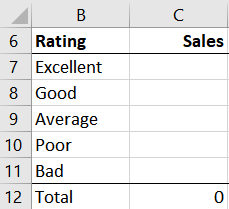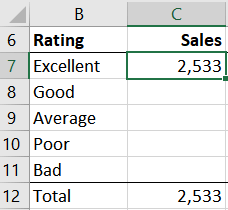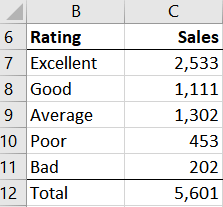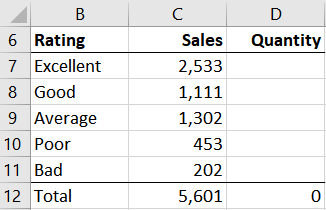## Sum a Column Based on Values in Another

In this post, we’ll learn how to add up a column of numbers based on the values in another column. For example, we are trying to analyze product sales based on average customer rating. That is, customers rate our products on a scale of 1 to 10, and so each product has an average rating such as 9.8, 7.2, 6.1, and so on. We would like to compute the sales totals for products rated from 9 to 10, and 7 to 8, and so on.

Just as with anything in Excel, there are multiple ways to accomplish this. But, I wanted an opportunity to talk about how the SUMIFS function supports the use of comparison operators, and this is a great example. And, since COUNTIFS also supports comparison operators, we may as well compute the number of products in each group as well.

The idea for this post came out of an email exchange with Eileen, who asked a question about adding a fee column based on the results in score column. Thanks Eileen!

## Objective

Before we jump right into the formulas, let’s take a quick look at our data so we can be clear about our goal. Our data includes the product ID, the average rating, and the total sales for the period. The data is stored in a table named Table1 … like this:We are trying to compute sales for each product group. “Excellent” products are rated 9 to 10, “Great” products are 7 to 8, “Average” products are 5 to 6, “Poor” products are 3 to 4, and “Bad” products are 1 to 2. Our summary should basically look like this:Now that we can see our endgame, let’s connect the dots and use SUMIFS and COUNTIFS. I’ve prepared a video and written narrative that provide the details.

## Narrative

We’ll use SUMIFS and COUNTIFS to compute our summary values. Let’s just take them one at a time.

## SUMIFS

Basically, the SUMIFS function is designed to add up a column of numbers, but, include only those rows that meet one or more conditions. Each condition is defined by a pair of arguments. Here is the basic syntax:

`=SUMIFS(sum_range, criteria_range1, criteria1, ...)`

Where:

• sum_range is the column of numbers to add
• criteria_range1 is the first criteria column
• criteria1 is the first criteria value
• … additional pairs of arguments

I’ve come up with a little narrative that helps me keep the function arguments straight, and hopefully, it can help you too. Basically, each “this” in the following narrative represents an argument: “Add up this column of numbers, but only include those rows where this column is equal to this value.”

Let’s see if we can apply this function to our summary report. Here is the summary report structure:Let’s take it one step at a time, and start by writing a formula in C7 that adds up the sales column, but only includes products with a rating equal to 9. This isn’t exactly what we want (we really want greater than 9, not equal to 9), but it will help us get the basic arguments into the function.

The data table is named Table1. So, using our narrative sounds like this “add up the Sales column, but only include those rows where the Rating column is equal to 9.”  Thus, our first formula looks like this:

`=SUMIFS(Table1[Sales], Table1[Rating],9)`

We hit Enter, and get 0. Why? Because there are no products with a rating equal to 9. So, how do we update the formula to include all products with a rating of 9 or more? Here is the answer and the entire point of this post:

• The SUMIFS function supports comparison operators in criteria value arguments

Said more simply: we can use greater than and less than logic. Greater than is represented by the > comparison operator, and less than uses the < operator. Greater than or equal to is like this >=, and less than or equal to like this <=.

So, we can make one small tweak to our formula by including the greater than or equal to operator >= in the criteria argument. Now, this all needs to be enclosed in quotes, so, the updated version of the formula is here:

`=SUMIFS(Table1[Sales], Table1[Rating], ">=9")`

We hit Enter and this time … yes, it worked!So far, Excellent sums all products with a rating that is greater than or equal to 9.

Note: in practice, I’d probably enter the value 9 into a cell rather than in the formula, and then update the formula to reference the cell. For example, if 9 was entered in cell A1, the updated formula would be: =SUMIFS(Table1[Sales], Table1[Rating], “>=”&A1)

Now, let’s think about the next category: Good. This needs to include products with ratings of 7 and 8. But, let’s be more precise. It needs to include products with a rating that is greater than or equal to 7 and less than 9. Make sense? Remember, our ratings include decimals, so we need to handle ratings of 7.1 and 8.5, etc. So, the formula for C8 is:

`=SUMIFS(Table1[Sales],Table1[Rating],">=7",Table1[Rating],"<9")`

Note: we referenced the Rating column twice. Can we do that? Yes! And, the SUMIFS function uses AND logic, so, all conditions must be true to be included in the sum. If you need to use OR logic instead, check out this post.

And we just continue like that to complete the summary. For example, the formula for Average products is:

`=SUMIFS(Table1[Sales],Table1[Rating],">=5",Table1[Rating],"<7")`

And, so on until we complete our summary:Now, all that remains is to compute the Quantity column:For that, we’ll use the COUNTIFS function.

## COUNTIFS

COUNTIFS basically works the same as SUMIFS, but it returns the count of the number of rows that satisfy the condition/s. As there is no column of numbers to add, we just use pairs of arguments to define the condition/s (there is no sum_range argument).

So, to compute the number of Excellent products, we would use this in D7:

`=COUNTIFS(Table1[Rating],">=9")`

Then, Good products in D8:

`=COUNTIFS(Table1[Rating],">=7",Table1[Rating],"<9")`

And so on down the summary. The finished summary is here:Yay … we did it! And that is how to sum (and count) a column of numbers based on the values in another column.

The formulas are provided in this sample file: ComparisonOperators.xlsx

Posted in ,### Jeff Lenning

I love sharing the things I've learned about Excel, and I built Excel University to help me do that. My motto is: Learn Excel. Work Faster.

### Stay Connected

If you'd like to be notified when I write a new Excel article, enter your name and email and click SUBSCRIBE. You can unsubscribe anytime, and I will never sell your email address.

### Want to learn Excel?

Our Campus Pass includes access to our entire Undergrad and Masters catalog. Gamification ensures it is the most fun you can have learning Excel :)

### 11 Comments

1.Robert Clark on May 23, 2019 at 8:02 am

Couldn’t something similar have been done with a pivot table using grouping on the rating, starting at 1.0, ending at 10.0, with an increment of 2?

•Jeff Lenning on May 23, 2019 at 8:12 am

Absolutely Robert, yes! My goal for this post was to demonstrate the fact that SUMIFS supports comparison operators. As to the specific report presented, yes PT would be another option. And that is one of the great things about Excel … there are usually multiple solutions 🙂

•Robert Clark on May 23, 2019 at 8:17 am

Thanks for confirmation. I also picked up that I need to built a quote string if I am comparing with a cell.

2.Leroy Bottomley on May 23, 2019 at 9:45 am

Is there anything like a sumifs version of Vlookup where you can specify multiple criteria from multiple columns in the table?
if column1 = A & column2 = 4 return value from column 3

3.Jean-Sébastien Quesnel on May 23, 2019 at 9:49 am

That’s great but is there a way to avoid putting numbers in the formulas?

I tried to replace the numbers (3,5,7,9) with cell references but it doesn’t seem to work. It works only without comparison operators.

•Jean-Sébastien Quesnel on May 23, 2019 at 10:06 am

Sorry, I only watched the video and didn’t read. I found the answer elsewhere and when I came back to update my comment, I saw it on your page.

The comparison operators have to be enclosed in quotes alone and the cell reference concatenated.

•Jeff Lenning on May 23, 2019 at 10:08 am

Great, glad you got it!
Thanks
Jeff

4.Ed on October 21, 2020 at 7:54 am

SUMIF question. I have this formula which works fine. =SUMIF(E4:E319,”>.2″,C4:C319). However, I would like the “>.2” part of the formula to be in a cell where you can change the value from .2 to whatever you would like. Is that possible?

Thanks in advance!

5.Ranjit singh on January 17, 2021 at 11:07 pm

I have a table name mail traffic in shéet1 where I have have headings: arl No. , Mail no., Mail type ( Urgent or Normal) and Number of mails , Date .Now
and I would like to make a table on Sheet2 basis on data in sheet1, where where I want to calculate mail count in one coloum and total no. Of perticular type mail in another coloum i.e total urgent mail and total Normal mail .will you please help to resolve

6.james wolter on February 15, 2021 at 11:11 am

This worked out PERFECTLY for what I needed to accomplish! Your video was straight forward and easy to follow!

### Learn by Email

###### Subscribe to Blog (free)
!
!
Something went wrong. Please check your entries and try again.